High School Physics : Newton's Laws

Example Questions

← Previous 1 3 4 5

Example Question #1 : Understanding Newton's First Law

Which of these is NOT an example of inertia?

A child riding a merry-go-round lets go and is thrown off the ride

A construction worker pushes a metal box and notices it is harder make the box start moving than it is to push it at a constant velocity

A passenger is thrown forward when his car collides with a wall

A satellite rotating in outer space will continue to spin at roughly the same rate

The force a hammer exerts on a nail is the same as the force the nail exerts on the hammer

The force a hammer exerts on a nail is the same as the force the nail exerts on the hammer

Explanation:

Newton's first law says that an object in motion will remain in motion in the same direction unless acted upon by an outside force; an object at rest will remain at rest unless acted upon by an outside force.

In the example of the car hitting the wall, the passenger continues travelling at the same speed that the car was moving directly before impact. He does not stop his forward motion until an outside force (his seatbelt) stops him.

In the example of the satellite, the rotation of the satellite can only change rate if an outside force interferes. Because there are no forces to affect the satellite's spinning, it will continue to do so as per Newton's first law.

In the example of the construction worker, the box was at rest and therefore resisted any change to being at rest. Once it is in motion, it will continue in motion in the same direction. This principle is the same reason why static coefficients of friction are generally greater than kinetic coefficients of friction.

In the example of the child on the merry-go-round, the important part of Newton's first law to recall is that objects will remain in motion in the same direction. The rotational motion of the child will result in a constantly changing velocity in the direction tangent to the edge of the ride. Once the child lets go, she will move in a straight line directly off of the ride.

The hammer and nail example illustrates Newton's third law, but has no bearing on the principle of inertia.

Example Question #1 : Newton's Laws

While pushing a very heavy box, Derek notices that it was much harder for him to get the box to start moving than it was for him to get the box to continue to move once it had started. This is an example of which mechanics principle?

Law of frictional force

Newton's first law

Newton's third law

Law of universal gravitation

Newton's second law

Newton's first law

Explanation:

This is an example of Newton's first law: an object at rest will remain at rest, and an object in motion will remain in motion in that direction, unless acted upon by an outside force.

Inertia is effectively nature's way of trying to avoid change. This explains why the box is hard to move while it is still; it requires change to get it to move from rest to moving. It is easier to continue motion when it is moving because it requires much less change to keep it moving in the same direction.

Mathematically, this principle dictates that the coefficient of static friction will always be greater than the coefficient of kinetic friction.

Example Question #3 : Newton's Laws

You are standing in a moving bus, facing forward, and you suddenly fall forward as the bus comes to an immediate stop.  What force caused you to fall forward?

Normal force due to your contact with the floor of the bus

Gravity

There is not a force learning to your fall

Force due to friction between you and the floor of the bus

There is not a force learning to your fall

Explanation:

While the bus in moving forward, you move forward with the bus at the same velocity.

When the bus stops, your body continue to move forward with the original velocity at which you had been traveling. Since you are not attached to the bus, you will not stop at the same rate as the bus and will fall forward as your body continues to move.

This is the reason why seatbelts are so important as it keeps you fixed to the car so that you undergo the same forces that are acting on the car.

Example Question #4 : Newton's Laws

A truck is traveling horizontally to the left.  On the bed of the truck is a crate.  When the truck starts to slow down, the crate on the (frictionless) truck bed starts to slide.  In what direction could the net force be on the crate?

Straight down (because of gravity)

Horizontal and to the left.

No direction.  The net force is zero.

Horizontal and to the right.

Straight up (the normal force)

No direction.  The net force is zero.

Explanation:

While the truck in moving forward, the carte moves forward with the truck at the same velocity.

When the trucks stops, the crate continues to move forward with the original velocity at which it had been traveling since there is no friction to stop the crate from moving. Since the crate is not attached to the truck, it will not stop at the same rate as the truck and will slide toward the cab of the truck as the truck slows down.

This is the reason why tie-downs are so important as it keeps objects fixed to the truck so that they undergo the same forces that are acting on the truck.

Example Question #5 : Newton's Laws

Conceptual

Which of these is NOT an example of inertia?

A child riding a merry-go-round lets go and is thrown off the ride

A passenger is thrown forward when his car collides with a wall

The force a hammer exerts on a nail is the same as the force the nail exerts on the hammer

A satellite rotating in outer space will continue to spin at roughly the same rate

A construction worker pushes a metal box and notices it is harder make the box start moving than it is to push it at a constant velocity

The force a hammer exerts on a nail is the same as the force the nail exerts on the hammer

Explanation:

Newton's first law says that an object in motion will remain in motion in the same direction unless acted upon by an outside force; an object at rest will remain at rest unless acted upon by an outside force.

In the example of the car hitting the wall, the passenger continues travelling at the same speed that the car was moving directly before impact. He does not stop his forward motion until an outside force (his seatbelt) stops him.

In the example of the satellite, the rotation of the satellite can only change rate if an outside force interferes. Because there are no forces to affect the satellite's spinning, it will continue to do so as per Newton's first law.

In the example of the construction worker, the box was at rest and therefore resisted any change to being at rest. Once it is in motion, it will continue in motion in the same direction. This principle is the same reason why static coefficients of friction are generally greater than kinetic coefficients of friction.

In the example of the child on the merry-go-round, the important part of Newton's first law to recall is that objects will remain in motion in the same direction. The rotational motion of the child will result in a constantly changing velocity in the direction tangent to the edge of the ride. Once the child lets go, she will move in a straight line directly off of the ride.

The hammer and nail example illustrates Newton's third law, but has no bearing on the principle of inertia.

Example Question #6 : Newton's Laws

While pushing a very heavy box, Derek notices that it was much harder for him to get the box to start moving than it was for him to get the box to continue to move once it had started. This is an example of which mechanics principle?

Newton's first law

Newton's second law

Law of frictional force

Newton's third law

Law of universal gravitation

Newton's first law

Explanation:

This is an example of Newton's first law: an object at rest will remain at rest, and an object in motion will remain in motion in that direction, unless acted upon by an outside force.

Inertia is effectively nature's way of trying to avoid change. This explains why the box is hard to move while it is still; it requires change to get it to move from rest to moving. It is easier to continue motion when it is moving because it requires much less change to keep it moving in the same direction.

Mathematically, this principle dictates that the coefficient of static friction will always be greater than the coefficient of kinetic friction.

Example Question #1 : Understanding Newton's First Law

In the absence of an external force, a moving object will

Slow down and eventually come to a stop

Move with constant velocity

Stop immediately

Go faster and faster

Move with constant velocity

Explanation:

According to Newton’s First law, an object in motion will remain in motion until there is an external force acting on this.  This is observable in the real world as marbles will continue to roll until they are slowed down by friction or stopped by a wall or a person or some other external force.

Example Question #1 : Understanding Newton's Second Law

Which of the following is not a part of Newton's second law?

Force

Mass

Displacement

Acceleration

Displacement

Explanation:

Newton's 2nd law states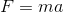. Therefore, all we need is a force, a mass, and an acceleration!

Example Question #2 : Understanding Newton's Second Law

A block is pushed withnewtons of force. What other information do we need in order to find the acceleration of the block?

Initial velocity

Mass

Work done on the block

Acceleration due to gravity

Final velocity

Mass

Explanation:

Newton's second law states that.

If we know the force,, then we only need to know the mass,, in order to find acceleration.

Example Question #3 : Understanding Newton's Second Law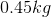orange falls from a tree. What is the force of gravity on the orange?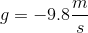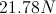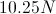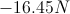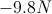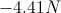Explanation:

Newton's second law states that:We are given the mass of the orange and the acceleration; since we are looking at the force due to gravity, the acceleration will be the acceleration due to gravity. Use these given values to calculate the force.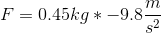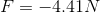Keep in mind that the force will be negative, since gravity acts in the downward direction.

← Previous 1 3 4 5